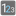• # Don't understand sorting algorithm

## Question related to missionBigger Together

My code:

```def bigger_together(array):

def convert(ar):
length = len(str(max(ar)))
return [(elem, length - len(elem)) for elem in map(str, ar)]

def get_number(ar, r):
return int(''.join(next(zip(*sorted(convert(ar), key=lambda x: x.ljust(x, '0'), reverse=r)))))

return get_number(array, True) - get_number(array, False)
```

This works correctly on a few examples, and on the rest incorrectly. I can not understand what key I need to use for sorting numbers. I think need to combine the length and number value. But I don’t guess how.

On example:

for Extra 2 test I get two numbers: 3232212 and 1222332. But I need get 3322212 and 1222323.35